# Midterm 3 Preparation and Sample Questions

This chapter contains several sections that will help prepare for the midterm exam:

# Midterm Three Composition

The third midterm will be composed of fifteen questions covering chapters 7 to 9. Twelve questions will be algebra questions, and three questions will be word problems.

• Questions 1–4 will be drawn from Chapter 8: Rational Expressions
• 8.1 Reducing Rational Expressions
• 8.2 Multiplication and Division of Rational Expressions
• 8.3 Least Common Denominators
• 8.4 Addition and Subtraction of Rational Expressions
• 8.5 Reducing Complex Fractions
• 8.6 Solving Complex Fractions
• 8.7 Solving Rational Equations
• Questions 5–8 will be drawn from Chapter 9: Radicals
• 9.1 Reducing Square Roots
• 9.2 Reducing Higher Power Roots
• 9.4 Multiplication and Division of Radicals
• 9.5 Rationalizing Denominators
• 9.6 Radicals and Rational Exponents
• 9.8 Radicals of Mixed Index
• 9.9 Complex Numbers (Optional)
• Questions 9–12 will be drawn from Chapter 10: Quadratics
• 10.1 Solving Radical Equations
• 10.2 Solving Exponential Equations
• 10.3 Completing the Square
• 10.4 The Quadratic Equation
• 10.5 Solving Quadratic Equations Using Substitution
•  10.6 Graphing Quadratic Equations—Vertex and Intercept Method
• 10.7 Quadratic Word Problems: Age and Numbers
• 10.8 Construct a Quadratic Equation from its Roots
• Questions 13–15 will be three word problems drawn from:
• 8.8 Rate Word Problems: Speed, Distance and Time
• 9.10 Rate Word Problems: Work and Time
• 10.7 Quadratic Word Problems: Age and Numbers

Students will be allowed to use MATQ 1099 Data Booklets & Glossaries for both Midterms and the Final Exam.

# Midterm Three Review

## Chapter 7: Rational Expressions (Exam Type Questions)

In the problems below, perform the indicated operations and simplify.

1.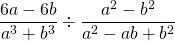2.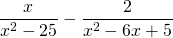3.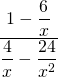Solve for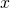.

1.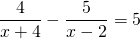## Chapter 8: Radicals (Exam Type Questions)

Questions 5–6 are for review only and will not show up on exams.

1.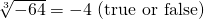2.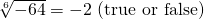Questions similar to 7–13 will show up on exams.

For questions 7–10, simplify each expression.

1.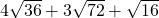2.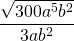3.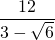4.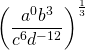For questions 11–13, solve for.

1.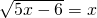2.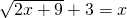3.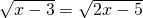## Chapter 9: Quadratics (Exam Type Questions)

Use the quadratic discriminant to determine the nature of the roots of each equation.

1.1.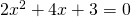2.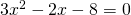For the questions below, find the solution set by any convenient method.

1.1.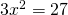2.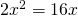2.1.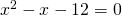2.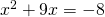3.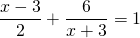4.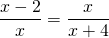## Chapter 7–9 Word Problems (Exam Type Questions)

1. The length of a rectangle is 3 cm longer than twice the width. If the area of the rectangle is 65 cm2, find its length and width.
2. Find three consecutive even integers such that the product of the first and the second is 68 more than the third integer.
3. A boat cruises upriver for 8 hours and returns to its starting point in 6 hours. If the speed of the river is 4 km/h, find the speed of this boat in still water.
4. The base of a right triangle is 8 m longer than its height. If the area of the triangle is 330 m2, what are its base and height measurements?

<a class=”internal” href=”/intermediatealgebraberg/back-matter/midterm-3-prep-answer-key/”>Midterm 3: Prep Questions Answer Key